# Fast R-CNN | ML

Before discussing Fast R-CNN, let’s look at the challenges faced by R-CNN

• The training of R-CNN is very slow because each part of the model such as (CNN, SVM classifier, bounding box) requires training separately and cannot be paralleled.
• Also, in R-CNN we need to forward pass every region proposal through the Deep Convolution architecture (that’s up to ~2000 region proposals per image). That explains the amount of time taken to train this model
• The testing time of inference is also very high. It takes 49 seconds to test an image in R-CNN (along with selective search region proposal generation).

Fast R-CNN works to solve these problems. Let’s look at the architecture of Fast R-CNN

First, we generate the region proposal from selective search algorithm. This selective search algorithm generates up to approximately 2000 region proposal. These region proposals (RoI projections) combines with input image passed into a CNN network. This CNN network generates the convolution feature map as output. Then for each object proposal, a Region of Interest (RoI) pooling layer extracts the feature vector of fixed length for each feature map. Every feature vector is then passed into twin layers of softmax classifier and Bbox regression for classification of region proposal and improve the position of the bounding box of that object.

CNN Network of Fast R-CNN

Fast R-CNN is experimented with three pre-trained ImageNet networks each with 5 max pooling layer and 5-13 convolution layers (such as VGG-16). There are some changes proposed in these pre-trained network, These changes are:

• The network is modified in such a way that it two inputs the image and list of region proposals generated on that images.
• Second, the last pooling layer (here (7*7*512)) before fully connected layers need to be replaced by region of interest (RoI) pooling layer.
• Third, the last fully connected layer and softmax layer is replaced by twin layers of softmax classifier and K+1 category-specific bounding box regressor with a fully connected layer.

This CNN architecture takes image (size = 224 x 224 x 3 for VGG-16) and its region proposal and outputs the convolution feature map (size = 14 x 14 x 512 for VGG-16).

Region of Interest (RoI) pooling: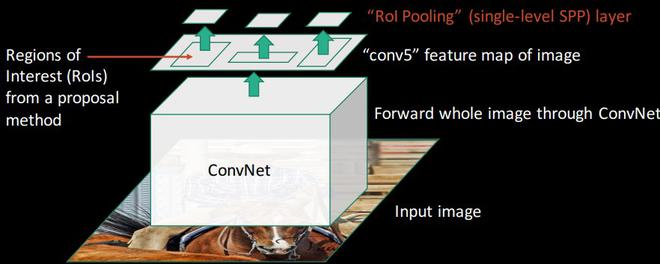RoI pooling is the novel thing that was introduced in Fast R-CNN paper. Its purpose is to produce uniform, fixed-size feature maps from non-uniform inputs (RoIs).
It takes two values as inputs:

• A feature map obtained from previous CNN layer (14 x 14 x 512 in VGG-16).
• An N x 4 matrix of representing regions of interest, where N is a number of RoIs, the first two represent the coordinates of upper left corner of RoI and other two represent the height and width of RoI denoted as (r, c, h, w).

Let’s consider we have 8*8 feature maps, we need to extract an output of size 2*2. We will follow the steps below.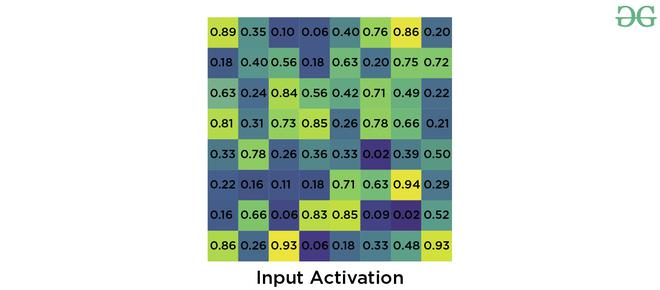• Suppose we were given RoI’s left corner coordinates as (0, 3) and height, width as (5, 7).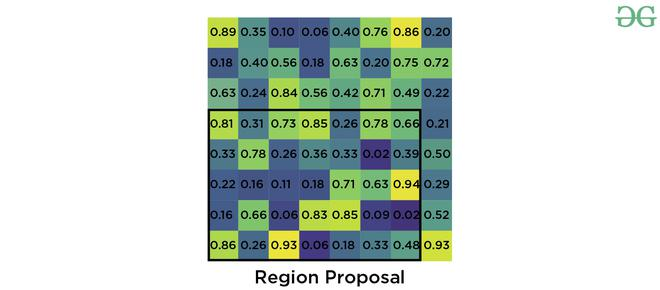• Now if we need to convert this region proposal into 2 x 2 output block and we know that the dimensions of the pooling section does not perfectly divisible by output dimension. We take pooling such that it is fixed into 2 x 2 dimensions.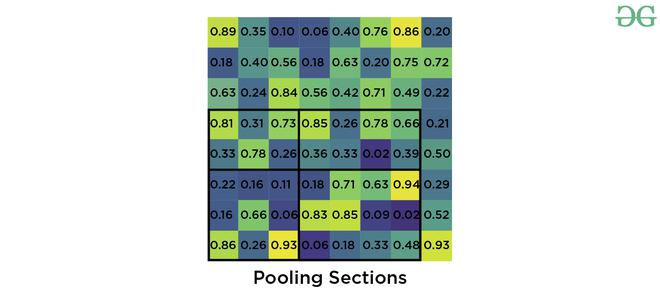• Now we apply max pooling operator to select the maximum value from each of the region that we divided into.

Training and Loss : First we take each training region of interest labeled with ground truth class u and ground truth bounding box v. Then we take the output generated by softmax classifier and bounding box regressor and apply loss function on them. We defined our loss function such that it takes into account both the classification and bounding box localization. This loss function is called multi-task loss. This is defined as follows: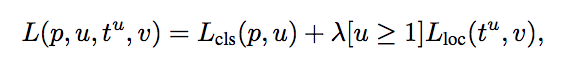where Lcls  is classification loss, Lloc is localization loss. lambda is balancing parameter and u is a function (the value of u=0 for background, otherwise  u=1) to make sure that loss is only calculated at when we need to define bounding box. Here, Lcls is the log loss and Lloc  is defined as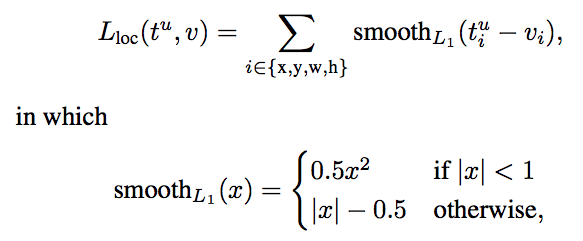Results and conclusion:

• Fast R-CNN provided state-of-the-art mAPs on VOC 2007, 2010 and 2012 datasets.
• It also improves detection time (84 vs 9.5 hrs) and training time (47 vs 0.32 seconds) considerably.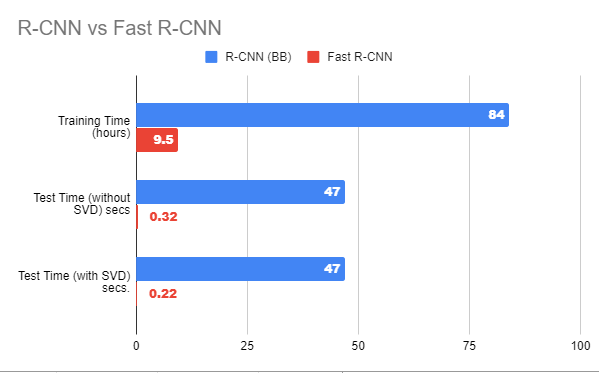Advantages of Fast R-CNN over R-CNN

• The most important reason that Fast R-CNN is faster than R-CNN is because we don’t need to pass 2000 region proposals for every image in the CNN model. Instead, the convNet operation is done only once per image and feature map is generated from it.
• Since, the whole model is combined and trained in one go. So, there is no need for feature caching. That also decreases disk memory requirement while training.
• Fast R-CNN also improves mAP as compare to R-CNN on most of the classes of VOC 2007, 10 and 12 datasets.

References:

My Personal Notes arrow_drop_upCheck out this Author's contributed articles.

If you like GeeksforGeeks and would like to contribute, you can also write an article using contribute.geeksforgeeks.org or mail your article to contribute@geeksforgeeks.org. See your article appearing on the GeeksforGeeks main page and help other Geeks.

Please Improve this article if you find anything incorrect by clicking on the "Improve Article" button below.

Article Tags :
Practice Tags :

Be the First to upvote.

Please write to us at contribute@geeksforgeeks.org to report any issue with the above content.